# Letters

January 2000

I recently noticed a curious fact about the number nine and the result of multiplying it. Could you provide an explanation for this beyond "coincidence"? The curious fact is this:

If you multiply any number from 1 through 31 by nine the result is a number that when the digits are added either equals nine or results in two nines. For example, 9 x 2 = 18; and 9 x 31 = 279.

When you continue multiplying 9 by larger and larger numbers a pattern appears to emerge.

In the 30's, only one number, 32, does not result in a sum that supports this phenomenon. 32 x 9 = 288. But 33 x 9 = 297.

In the 40's, only two numbers do not work, 42 and 43.

In the 50's, three numbers don't work, 52, 53, 54.

In the 60's, four numbers don't work, 62-65.

As you might expect, in the 70's five numbers, 72-76, don't work.

I expect this pattern goes on and then perhaps doubles back. While it has been an amusing dinner time conversation, I have not checked it out.

Is this explicable?

Jon Metropoulos

It's simpler to analyse this problem if you repeat the digit addition whenever it fails, and replace all 9's by zeroes whenever they appear. All multiples of 9 will then yield the answer 0.

So,

 32 x 9 = 288 fails but on summing the digits... 288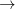18 fails but on summing the digits... 189 fails but on replacing 9 by 0... 90 success!

This calculation actually succeeds in calculating the remainder when a number is divided by 9. So for example...

 764320 202

and 7643/9 = 849 remainder 2.

Using this modified procedure (known to accountants as ’casting out nines’), you can get a quick check on the accuracy of any sum by doing the equivalent sum with all nines cast out (i.e. in arithmetic modulo 9).

So... does 26 + 389 + 35 + 36 = 498?

No! because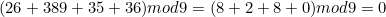but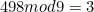I’ll leave proving why repeatedly taking the sum of the digits is equivalent to finding the remainder modulo 9 to you. It all depends on noticing that any number N expressed in base 10 can be written as: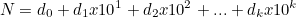where the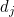are the digits of the number, and noting that 10 mod 9 = 1!

(Note that 9 is not the only digit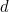where 10 mod= 1! Is there another divisibility test lurking here somewhere?)

Going back to your original definition of whether a number works or not, it would make sense for a pattern to emerge for small numbers, but for it to get increasingly chaotic as the numbers get larger. Is this what you find?

Mike Pearson

James R. Foster

Since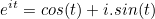where=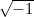andis any angle in radians, therefore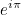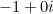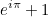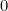(2)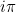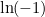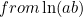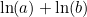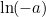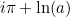(3)
Now, from equation 1 we have: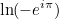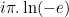From equation 2 we have: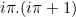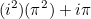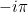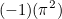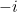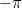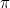or, keeping everything real...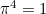.

This can be disproven by saying "oi, look, no it doesn't", but can anyone disprove this paradoxical proof?

The main problem is with the lines:-(2)(3)
which does not follow as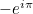means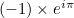rather than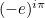. The second problem is that the logarithm of a complex number is not a well-defined function unless you specify its range more carefully. This is because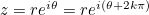for any integer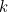. So:The correct deduction from (1) is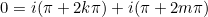for some integral values ofand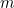. ...which is correct (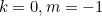for example)

Mike Pearson

## Mysterious sequence

Please could you help us solve a maths sequence we have been puzzled with for a while.

It's to find the Nth term of the following sequence:

0, 11, 28, 51, 80

Miss G. Cornish

This one yields to the method of differences...

   1   2   3   4   5  = n                                      0  11  28  51  80  = S(n)      \ / \ / \ / \ /                 11  17  23  29                     \ / \ / \ /                        6   6   6



Since we have a constant difference in the second row, a quadratic formula for the nth term will work - something like: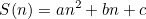Insert some values for n and S(n) and solve the resulting equations for a, b and c and the answer should drop out.

Alternatively, you could look for patterns in the factors.

Mike Pearson

## Waves

I am in the process of starting a piece of A-level coursework on mathematical equations of wave motion. I am hoping to focus on simple harmonic motion and I was hoping that you could provide me with a starting point on how to set up differential equations around s.h.m, enabling me to investigate waves to the fullest.

Amrit Summan

You will get a clear answer to this question if you simply refer to your A level mechanics text book.

## Gyroscopes

Can you provide a source for the battery-powered gyros you mention [in the Issue 7 article Galloping gyroscopes]?

Dwight Ufford

These were custom made in the University Engineering Lab and we think they would be quite expensive to manufacture. You could try the gyro car enthusiasts - they may be able to point you to a lower power version suitable for hand-held use.

## Constructive criticism?

"this is one of the worst websites ive ever been on ever and its boring and geeky and dull and stupid and im only on cos i was forced on it would never encourage someone in to mathss" [sic]

Anonymous Berkshire student